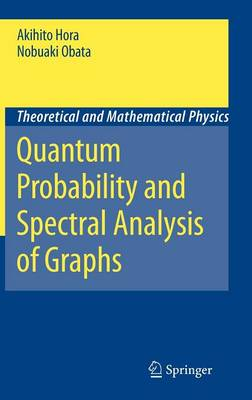•Quantum Probability and Spectral Analysis of Graphs - Theoretical and Mathematical Physics (Hardback)

(author), (author), (foreword)
£103.50
Hardback 371 Pages / Published: 02/05/2007
• We can order this

Usually dispatched within 3 weeks

This is the first book to comprehensively cover quantum probabilistic approaches to spectral analysis of graphs, an approach developed by the authors. The book functions as a concise introduction to quantum probability from an algebraic aspect. Here readers will learn several powerful methods and techniques of wide applicability, recently developed under the name of quantum probability. The exercises at the end of each chapter help to deepen understanding.

Publisher: Springer-Verlag Berlin and Heidelberg GmbH & Co. KG
ISBN: 9783540488620
Number of pages: 371
Weight: 758 g
Dimensions: 235 x 155 x 22 mm
Edition: 2007 ed.

MEDIA REVIEWS

From the reviews:

"It is a very accessible introduction for the non expert to a few rapidly evolving areas of mathematics such as spectral analysis of graphs ... . this monograph seems to be the first publication providing a synthesis of a very vast mathematical literature in these areas by giving to the reader a concise and self contained panorama of existing results ... . this book is important to the quantum probability community and emphasizes well many new applications of quantum probability to other areas of mathematics." (Benoit Collins, Zentralblatt MATH, Vol. 1141, 2008)